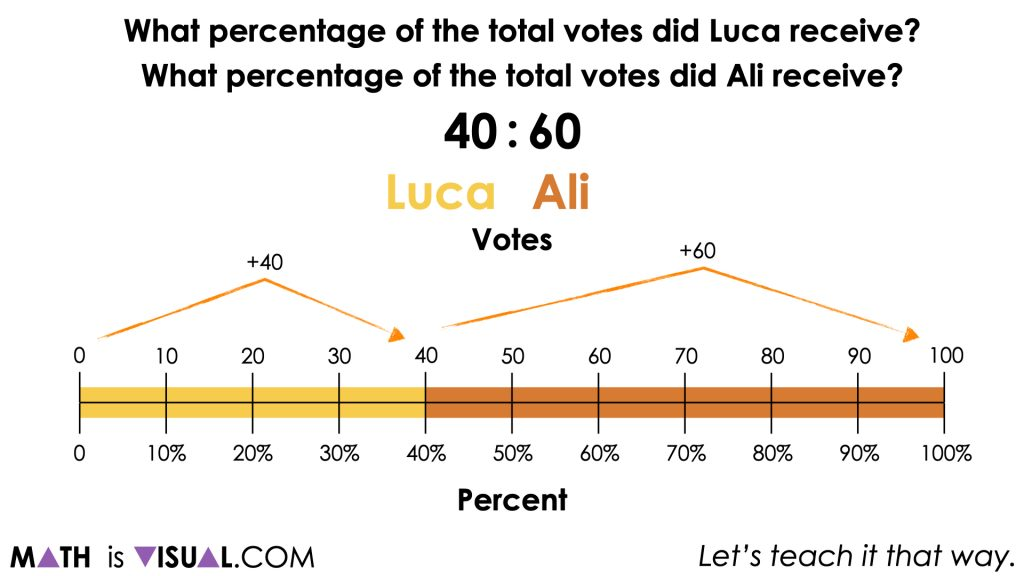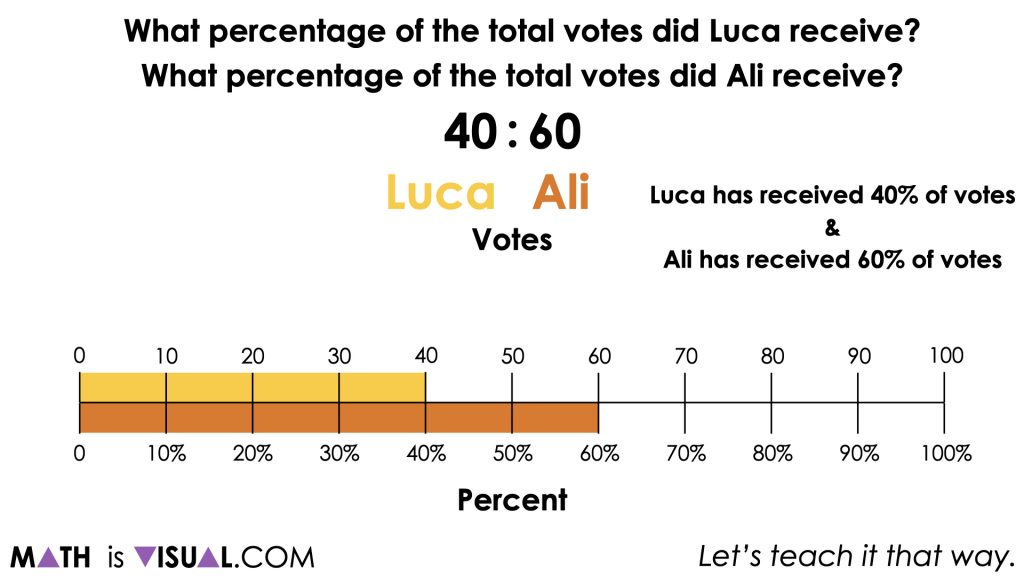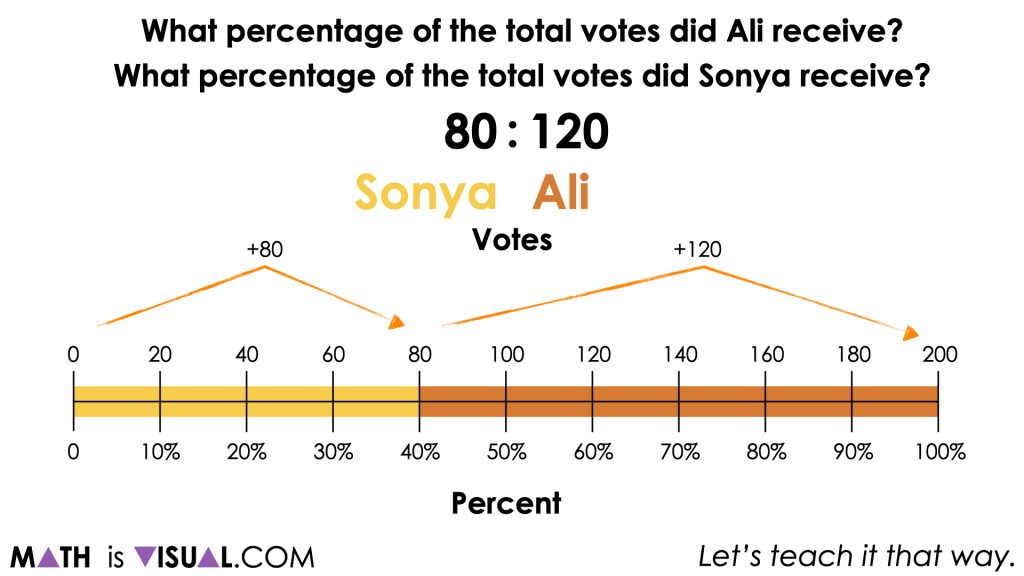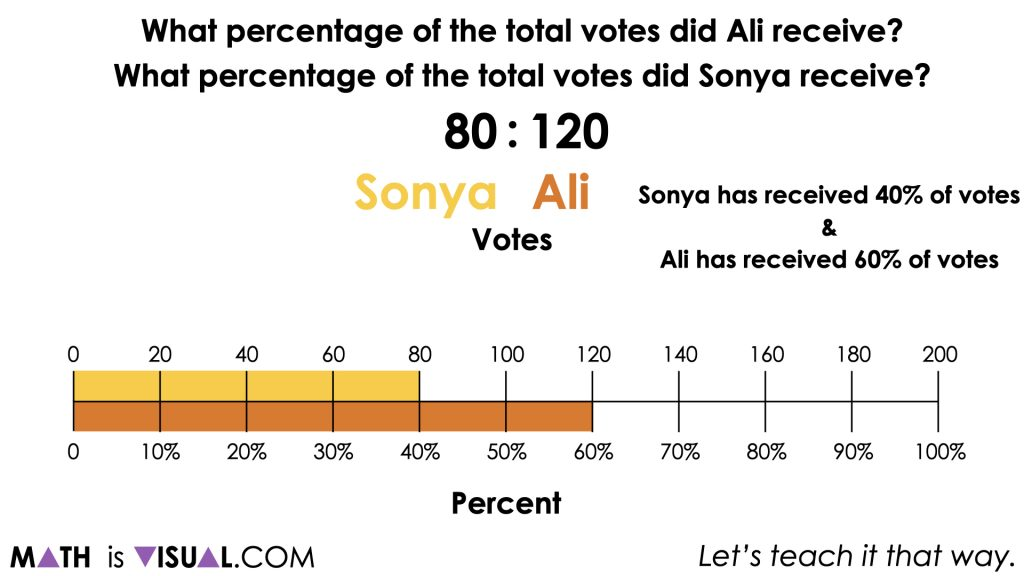# Representing Ratios As Percentages

Visual math talk prompts with context used to help emerge the writing and solving of one-step algebraic equations.

## In This Set of Math Visual Prompts…

Students will explore visual math talk prompts highlighting multiplicative comparison ratios represented as percentages using double number lines.

## Intentionality…

This set of visual math talk prompts is taken from the Math Talk section of Day 2 in the Make Math Moments Problem Based Unit called Voting Booth. The purpose of the Math Talk is to reinforce key concepts and big ideas from this problem based math unit including:

• A part of a whole represented as a percentage is a part-whole ratio whose whole is 100;
• A percentage is one of infinitely many equivalent part-whole ratios in a ratio relationship;
• A percentage can be used to scale in tandem and reveal an infinite number of equivalent ratios in a ratio relationship;
• Partitive division can be used to divide a percentage by 100 percent to reveal a rate for the proportional relationship.

Unsure about some of the terminology listed above?

## Preparing to Facilitate

Present the following multiplicative comparison ratios one at a time leveraging the context from Day 1 of the Voting Booth problem based unit where the two numbers in the ratio represent the number of votes. Encourage students to represent the following ratios on a double number line with votes as one quantity and percentage as the other.

Then, ask students to express the number of votes for each participant as a percentage of the total votes.

40 : 60

80 : 120

Three (3) additional prompts are shared in the Teacher Guide from Day 2 of the Voting Booth problem based math unit

Encourage students to utilize any tool of their choosing (other than a calculator) to assist them in this work. For example, a double number line could be beneficial to assist in completing this task. Since the sum of both quantities in this ratio represent 100% of the total votes, the bottom of the number line could span from 0 to 1 (or 0% to 100%) and the top of the number line could span from 0 to the sum of the parts. For example, in the second problem in the string, the top of the number line would span from 0 to 200 since 80 + 120 = 200.

## Visual Math Talk Prompt #1

The prompt builds off of the context from the first day of this problem based math unit where we ask students:

Some students may ask for clarification regarding how many people were a part of this vote. You can confirm that Luca and Ali were the only people involved in the vote and therefore the sum of their votes represents the total number of votes.

While students are free to leverage strategies and models of their choosing, you may ask students if you can represent their thinking on a double number line (or stacked bar model) similar to the following:This first prompt provides quite a low floor to allow all students to enter the problem and to reiterate or review what a percentage is and how they can be helpful when comparing two or more quantities like we see in this context.

After students arrive at the final answers of 40% and 60%, you can also line the bars up to make these quantities more explicit as you’ll see in the following screenshot.## Visual Math Talk Prompt #2

As you continue to play the video, you’ll see the second visual math talk prompt where we begin to raise the floor by asking the same question, except we have another vote involving Sonya who received 80 votes and Ali who received 120 votes.

As we did previously, we’ll allow students to solve using their own strategies and models, but encouraging a linear model when representing student thinking.Here, we can see that the total number of votes is 200, which can be represented as 100% and from there, we can partition to reveal that 80 votes represents 40% of the total votes and 120 votes represents the remaining 60%.It can be helpful to highlight that in the second vote, the number of votes received by each participant has doubled, yet the percentage of the whole remains the same. This is because 80:120 and 40:60 are equivalent ratios from the same proportional relationship.

## Want to Explore These Concepts & Skills Further?

Four (4) additional math talk prompts are available in Day 2 of the Voting Booth problem based math unit that you can dive into now.

Why not start from the beginning of this contextual 5-day unit of ratios and rates real world lessons from the Make Math Moments Problem Based Units page.

Did you use this in your classroom or at home? How’d it go? Post in the comments!

Math IS Visual. Let’s teach it that way.